Courses

# Feedback Characteristics

## 10 Questions MCQ Test Mock Test Series for Electrical Engineering (EE) GATE 2020 | Feedback Characteristics

Description
This mock test of Feedback Characteristics for GATE helps you for every GATE entrance exam. This contains 10 Multiple Choice Questions for GATE Feedback Characteristics (mcq) to study with solutions a complete question bank. The solved questions answers in this Feedback Characteristics quiz give you a good mix of easy questions and tough questions. GATE students definitely take this Feedback Characteristics exercise for a better result in the exam. You can find other Feedback Characteristics extra questions, long questions & short questions for GATE on EduRev as well by searching above.
QUESTION: 1

### In the system shown in figure below, the sensitivity of the closed loop transfer function w.r.t. parameter a is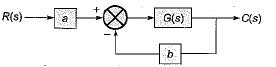Solution:

The closed loop transfer function is given by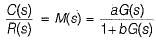∴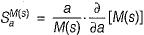Now,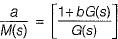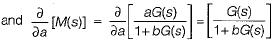∴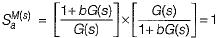QUESTION: 2

### For the system shown in figure below,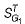is equal to (where, T = closed loop transfer function)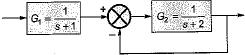Solution:

The CLTF is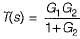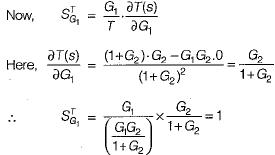QUESTION: 3

### In control system, the feedback

Solution:
QUESTION: 4

For the closed loop transfer function shown below, E(s)/R(s) is given by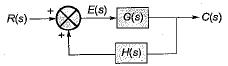Solution:

We have:   E(s) = R(s) + C(s) H(s) ...(i)

Also,   C(s) = E(s) G(s) ...(ii)
From (i) and (ii), we have:
E(s) = R(s) + E(s) G(s) H(s)
or, E(s) (1-G(s)H(s)] = R(s)
or,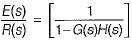QUESTION: 5

Due to positive feedback

Solution:
QUESTION: 6

Consider the following statements:
1. Closed loop system is less sensitive to variations in feedback path parameters than variations in forward path parameters.
2. Feedback can improve the stability or may be harmful to stability if it is not properly designed and applied.
3. Feedback controls the time response of the system by adjusting the location of poles.

Q. Which of the these statements are correct?

Solution: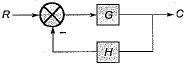For the closed loop control system shown above, let, H = C/R transfer function.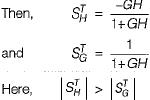Thus, closed loop system is more sensitive to variation in feedback path parameters than variation in forward path parameters. Hence statement-1 is false. Thus, statements 2 and 3 are only correct.

QUESTION: 7

An unity feedback control system has a transfer function of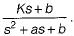The open loop transfer function of this system is

Solution: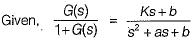∴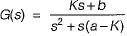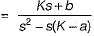QUESTION: 8

Assertion (A): Feedback in control system improves the time response of the system. ​
Reason (R): Time constant of an open loop system is less than the time constant of a closed loop system.

Solution:

Time constant of open loop system is more than that of closed loop system. Lesser is the time constant of the system, faster is its' time response.

QUESTION: 9

For the unity feedback system shown below, if the input to the system is a unit-step signal, then the integral square error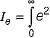(t) dt for infinite value of gain k will be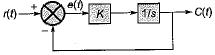Solution: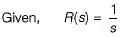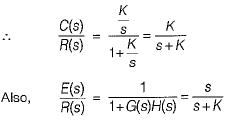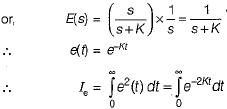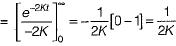Thus, when K = ∞, Ie = 0.

QUESTION: 10

Introduction of feedback to a system

Solution:

Introduction of feed back to a system will increase the stability if negative feedback is introduced while it will reduce the stability if positive feedback is introduced.